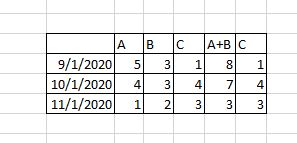# Formulas Using Measure and DimensionsHello, I am new to using Dundas. I had a question around formulas. I have uploaded an image for illustration. I have created a metric set table showing date as a row and commodity as a column (labeled A, B, C in example above). How can I create calculated columns such as A+B where column A and B are summed?

1 Like

Hello @patrick,

From the Metric Set(Data analysis panel) you can add a measure, click formula and write in the box \$A\$+\$B\$. This will create a new measure based on your formula.

1 Like

Hi Costin,

Thank you for the quick response. I looked through the documentation and still had some questions. On the rows in the data analysis panel I have “Date”, on the columns I have “Commodity”. A measure for volume is also in the table. To add A and B together in your example how do you reference the Volume for the dimension “Commodity” with and Attribute of A + Volume for the dimension “Commodity” with an Attribute of B.

Hello @patrick

Good question. If you will try it on a flat table this will work fine.
In your example, from what I’ve understood, you have a table which has columns with headers. I am not an expert in formulas, but I think the A+B formula will not work because I not know how you can refer only to Sales from A and Sales from B and the C “column” will not be affected. When you will create a formula based on Sales measure, this will take the “full” values without taking in consideration the split of the columns (A, B, C). i think it will just double the values from each column: Sales from Ax2, Sales from Bx2 etc.)

From my perspective, you should try to create these calculation into the DataCube(aggregation, calculated elements, queries etc.)

Hi Costin,

Thanks for the reply. I actually reached out to Dundas support on this issue. To create variables on the visual side where you filter based off dimensions you actually need to set it up as an if statement. Let’s say I have a dimension called Asset and I want to sum all the Volume where Asset is Generator your formula would be as follows:

if(\$Asset\$==“Generator”) return \$Volume\$; else return 0;

I am still figuring out the ways the new field can be incorporated across dashboards/metric sets

1 Like

Hello @patrick,

Good point! I will also check it on my end.
Thank you for the update!